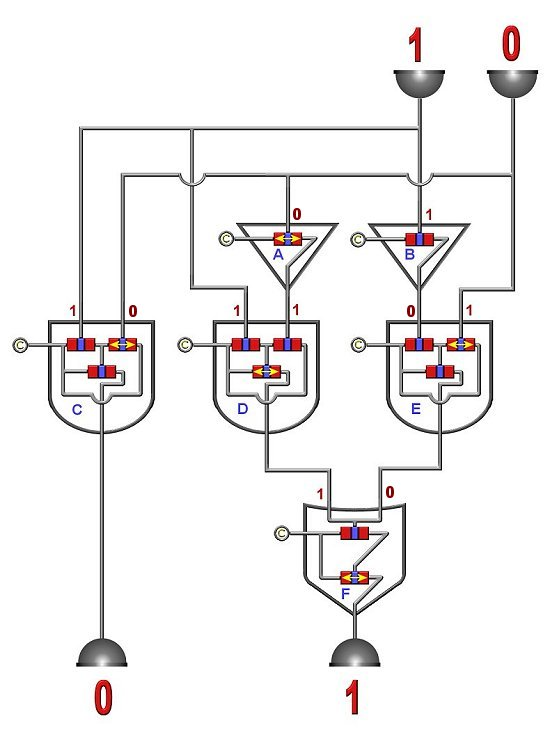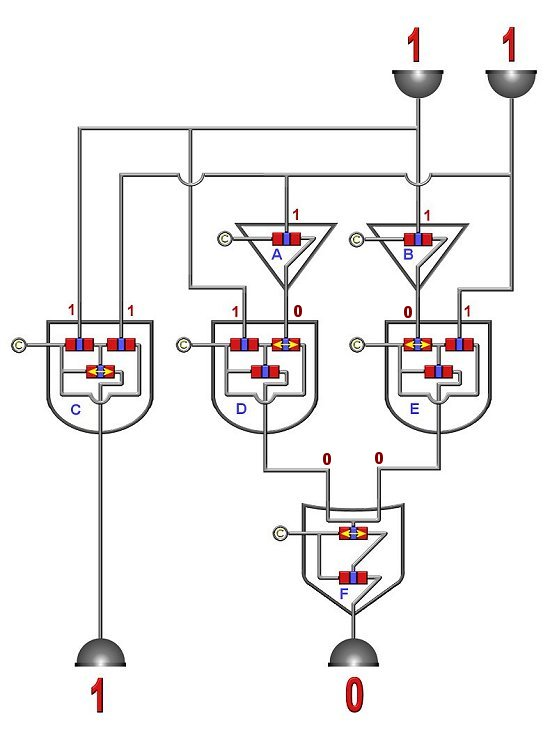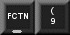### To understand logic gates you must understand the transistor used in the construction of gates. Without getting into a lot of physics, know that a transistor is made of three parts. This is shown in the above picture as the two red and one blue section. Most transitors have three wires and one wire would be attached to each of these sections. One of the most basic parts in a computer circuit is a clock. Now this clock doesn't really keep time (though it can) but is used to produce very fast, accurate pulses or signals. When I say fast, I mean it. Pulses can be anywhere from 1/100,000 of a second to 1/1,000,000,000 of a second. As humans we can't even imagine anything going that fast so it will suffice to say that computers are useful only because they can do everything very fast.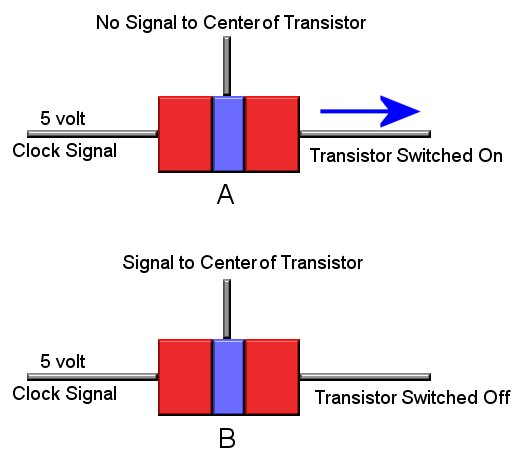### Back to our transistor. As can be seen above, one section of the transistor is connected to the clock. In most computer circuits this will be a 5 volt signal. In "A" of the above picture the transistor is "on" because there is NO signal to the center section of the transitor. So in other words when the clock sends the transistor a pulse the transistor will also send out a pulse from its output. Now notice in "B" of the picture the center section of the transistor is now recieving a signal. This in turn turns "off" the transistor. This might seem backwards, but in the world of logic circuits it works out nicely. It is very important that you understand the concept of a switching transistor to understand logic circuits. Make sure you understand the above before moving on to logic gates.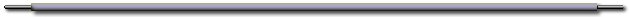### Logic Gates are the building blocks for all digital circuits. There are several of them, and we are going to take a look at the three most used. If you understand these three you will have no problems understanding the remaining ones as they are just extensions of these three. Logic gates are comprised of the type of transistors described above. Another concept we need to understand is the binary system. It is out of the scope of this page to teach binary math, but knowing that the binary systems contains two numbers: 1 and 0 will suffice. Binary numbers are the basis of all math functions performed by digital computers. Remember above when we discussed transistors and how they can be switched "on" or "off"? Well, in binary if the transistor is "off" then we consider that a "0". If the transistor is "on" then we consider that a "1". In fact this is the whole basis to digital. Everything that happens in the digital world can all be summed down to being either 1's or 0's, and nothing in between. On, Off, Yes, No, 1,0, no mediums, maybes, or any other numbers. That's it, that is the magic of digital.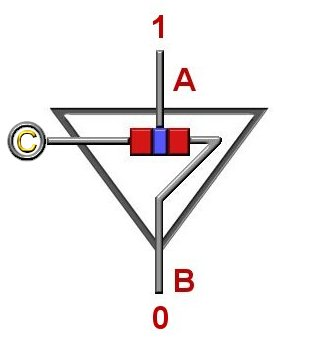### 0### An AND gate is another of the basic building block logic gate. An AND gate consists of three transistors, and as can be seen is more complicated than a NOT gate. Also notice that it's truth table is quite larger than the NOT's. As it's name and truth table implies only when the inputs (A,B) are 1 "AND" 1 will you get an output(C) of 1. The yellow arrow on the lower transitor shows that this transistor is "on". Since the two upper transistors are getting a signal they are both switched "off". If either one of them would be switched "on" then their output would send a signal to the lower transistor's center section which would switch the lower transistor "off" resulting in an ouput of 0. Make sure you understand why this is so.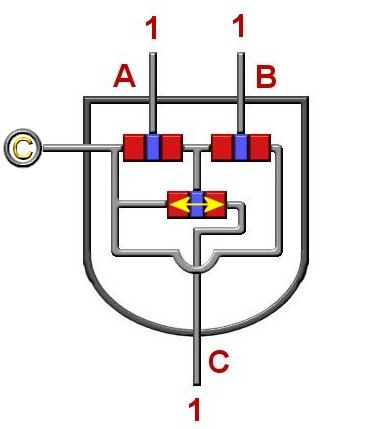### 1### Pictured below is an OR gate. As the name and the truth table implies, if input(A) "OR" input(B) is a 1 then the output(C) will be a 1. An OR gate consists of two transistors. In the example below input(A) is 1 and input(B) is a 0. Since one of the inputs is a 1 then the output(C) is a 1. Since one of the inputs is a 1 it sends a signal to the center of the upper transistor which turns it "off". Since the upper transistor is switched "off" no signal goes to the center part of the lower transistor which means that it is switched "on" which results in an output of 1.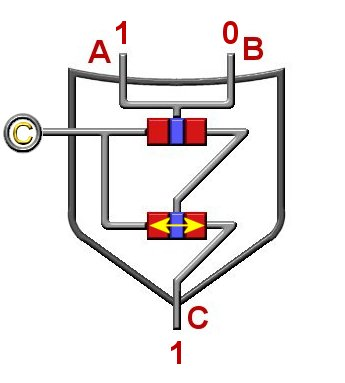### 1### Below are two adder circuits. See if you can follow the "bits" through. Hint: Any gate transistor that has a yellow double arrow is "on".